# 矿山围岩蠕变破坏过程数值模拟Numerical Simulation of Creep Failure Process of Mine Surrounding Rock

DOI: 10.12677/ME.2019.72027, PDF, HTML, XML, 下载: 437  浏览: 1,391

Abstract: Creep property of rock mass has a significant impact on the stability of underground constructions. On the basis of classical power-law creep model, the time-dependent creep behavior of rock is in-vestigated based on damage mechanics principle, in which the damage evolution is considered as a key factor dominating the accelerating creep and a damage-based constitutive law for tertiary creep is proposed. The maximum tensile strain criterion and the Mohr-Coulomb criterion are utilized as two damage thresholds to control the rock damage. Then the damage-based creep model is implemented using finite element method by MATLAB programming, a powerful PDE-based multiphysics modeling environment. The model is firstly validated by comparing the numerical results with the previously published experimental observation and then used to simulate the rock creep under uniaxial and biaxial compression. The model accurately reproduces the classic tri-modal behaviour (primary, secondary and tertiary creep) seen in laboratory creep (constant stress) experiments. So the fact shows that the rheological model is appropriate to predict the nonlinear creep failure of rocks, and the complex macroscopic time-dependent behavior can be mechanically explained by the material degradation (damage) at the mesoscale.

1. 引言

2. 蠕变损伤模型建立

2.1. 蠕变本构模型

$\epsilon ={\epsilon }_{\text{e}}+{\epsilon }_{\text{c}}$ (1)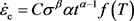(2)

B是比例因子， $\Delta H$ 是活化能，R是普适气体常数，T是温度。

${\stackrel{˙}{\epsilon }}_{\text{c}}=A{\sigma }^{\beta }\alpha {t}^{\alpha -1}$ (3)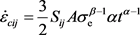(4)

${\sigma }_{\text{e}}=\left(\frac{1}{\sqrt{2}}\right){\left[{\left({\sigma }_{11}-{\sigma }_{22}\right)}^{2}+{\left({\sigma }_{33}-{\sigma }_{22}\right)}^{2}+{\left({\sigma }_{11}-{\sigma }_{33}\right)}^{2}+6\left({\sigma }_{12}^{2}+{\sigma }_{23}^{2}+{\sigma }_{13}^{2}\right)\right]}^{\frac{1}{2}}$ (5)

2.2. 损伤演化方程

${F}_{1}\equiv -{\sigma }_{3}-{f}_{t0}=0$${F}_{2}\equiv {\sigma }_{1}-{\sigma }_{3}\frac{1+\mathrm{sin}\theta }{1-\mathrm{sin}\theta }-{f}_{c0}=0$ (6)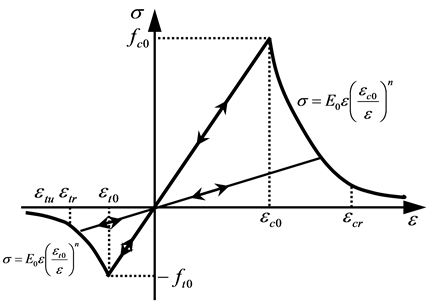Figure 1. Elastic damage constitutive law of element under uniaxial tensile and compressive stress

$E=\left(1-D\right){E}_{0}$ (7)

$D=\left\{\begin{array}{l}0\text{\hspace{0.17em}}\text{\hspace{0.17em}}\text{\hspace{0.17em}}\text{\hspace{0.17em}}\text{\hspace{0.17em}}\text{\hspace{0.17em}}\text{\hspace{0.17em}}\text{\hspace{0.17em}}\text{\hspace{0.17em}}\text{\hspace{0.17em}}\text{\hspace{0.17em}}\text{\hspace{0.17em}}\text{\hspace{0.17em}}\text{\hspace{0.17em}}\text{\hspace{0.17em}}\text{\hspace{0.17em}}\text{\hspace{0.17em}}\text{\hspace{0.17em}}\text{\hspace{0.17em}}{F}_{1}<0\text{\hspace{0.17em}}\text{\hspace{0.17em}}\text{and}\text{\hspace{0.17em}}\text{\hspace{0.17em}}{F}_{2}<0\\ 1-{|\frac{{\epsilon }_{t0}}{{\epsilon }_{1}}|}^{n}\text{\hspace{0.17em}}\text{\hspace{0.17em}}\text{\hspace{0.17em}}\text{\hspace{0.17em}}\text{\hspace{0.17em}}\text{\hspace{0.17em}}\text{\hspace{0.17em}}\text{\hspace{0.17em}}\text{\hspace{0.17em}}{F}_{1}=0\text{\hspace{0.17em}}\text{\hspace{0.17em}}\text{and}\text{\hspace{0.17em}}\text{\hspace{0.17em}}d{F}_{1}>0\\ 1-{|\frac{{\epsilon }_{c0}}{{\epsilon }_{3}}|}^{n}\text{\hspace{0.17em}}\text{\hspace{0.17em}}\text{\hspace{0.17em}}\text{\hspace{0.17em}}\text{\hspace{0.17em}}\text{\hspace{0.17em}}\text{\hspace{0.17em}}\text{\hspace{0.17em}}{F}_{2}=0\text{\hspace{0.17em}}\text{\hspace{0.17em}}\text{and}\text{\hspace{0.17em}}\text{\hspace{0.17em}}d{F}_{2}>0\end{array}$ (8)

3. 数值模拟

3.1. 数值模型验证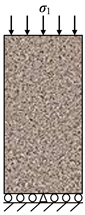Figure 2. Model boundary condition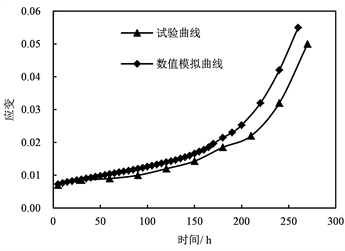(a) 邱贤德的蠕变试验结果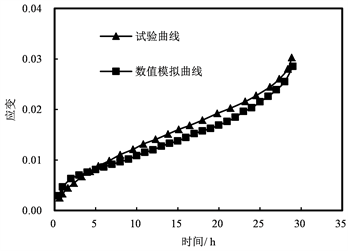(b) 杨春和的蠕变试验结果

Figure 3. Comparison between simulated creep curve and experimental creep curve

3.2. 蠕变特性数值模拟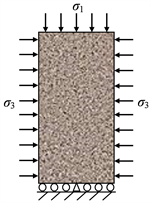Figure 4. Calculation modelTable 1. Physico-mechanical parameters of model specimens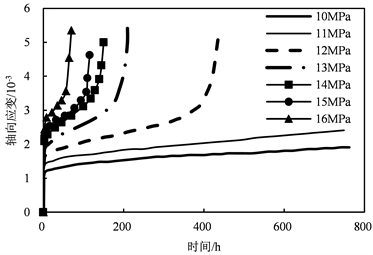Figure 5. Numerically obtained creep deformation curves under uniaxial compression creep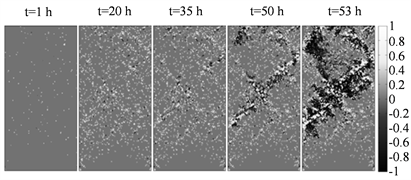Figure 6. Failure process of rock specimen under uniaxial compression creepFigure 7. Numerically obtained creep deformation curves under biaxial compression creep

4. 结论

  Diederichs, M.S. and Kaiser, P.K. (1999) Tensile Strength and Abutment Relaxation as Failure Control Mechanisms in Underground Excavations. International Journal of Rock Mechanics and Mining Sciences, 36, 69-96.https://doi.org/10.1016/S0148-9062(98)00179-X  Nara, Y., Takada, M., Mori, D., et al. (2010) Subcritical Crack Growth and Long-Term Strength in Rock and Cementitious Material. International Journal of Fracture, 164, 57-71. https://doi.org/10.1007/s10704-010-9455-z  张强勇, 向文, 江力宇, 等. 片麻状花岗岩热黏弹塑性损伤蠕变模型及应用研究[J]. 土木工程学报, 2017(8): 88-97.  Chen, Y.-L. and Azzam, R. (2007) Creep Fracture of Sandstones. Theoretical and Applied Fracture Mechanics, 47, 57-67. https://doi.org/10.1016/j.tafmec.2006.10.006  李永盛. 单轴压缩条件下四种岩石的蠕变和松弛试验研究[J]. 岩石力学与工程学报, 1995, 14(1): 39-47.  Shao, J.F., Zhu, Q.-Z. and Su, K. (2003) Modeling of Creep in Rock Materials in Terms of Material Degradation. Computers and Geotechnics, 30, 549-555. https://doi.org/10.1016/S0266-352X(03)00063-6  Cruden, D.M., Leung, K. and Masoumzadeh, S. (1987) A Technique for Estimating the Complete Creep Curve of a Sub-Bituminous Coal under Uniaxial Compression. International Journal of Rock Me-chanics and Mining Sciences & Geomechanics Abstracts, 24, 265-269. https://doi.org/10.1016/0148-9062(87)90181-1  徐卫亚, 杨圣奇, 谢守益, 等. 绿片岩三轴流变力学特性的研究(II): 模型分析[J]. 岩土力学, 2005, 26(5): 693-698.  曹文贵, 袁靖周, 王江营, 等. 考虑加速蠕变的岩石蠕变过程损伤模拟方法[J]. 湖南大学学报(自然科学版), 2013, 40(2): 15-20.  缪协兴, 陈至达. 岩石材料的一种蠕变损伤方程[J]. 固体力学学报, 1995(4): 343-346.  杨春和, 陈锋, 曾义金. 盐岩蠕变损伤关系研究[J]. 岩石力学与工程学报, 2002, 21(11): 1602-1604.  谢和平. 岩石混凝土损伤力学[M]. 徐州: 中国矿业大学出版社, 1990.  张强勇, 杨文东, 张建国, 等. 变参数蠕变损伤本构模型及其工程应用[J]. 岩石力学与工程学报, 2009, 28(4): 732-739.  佘成学. 岩石非线性黏弹塑性蠕变模型研究[J]. 岩石力学与工程学报, 2009, 28(10): 2006-2011.  Nadimi, S., Shahriar, K., Sharifzadeh, M. and Moarefvand, P. (2011) Triaxial Creep Tests and Back Analysis of Time-Dependent Behavior of Siah Bisheh Cavern by 3-Dimensional Distinct Element Method. Tunnelling and Underground Space Technology, 26, 155-162. https://doi.org/10.1016/j.tust.2010.09.002  Zhu, W.C. and Tang, C. (2004) Micromechanical Model for Simulating the Fracture Process of Rock. Rock Mechanics and Rock Engineering, 37, 25-56. https://doi.org/10.1007/s00603-003-0014-z  邱贤德, 姜永东, 阎宗岭, 等. 岩盐的蠕变损伤破坏分析[J]. 重庆大学学报, 2003, 26(5): 106-109.  Yang, C., Daemen, J.J.K. and Yin, J.H. (1999) Experimental Investigation of Creep Behavior of Salt Rock. International Journal of Rock Mechanics and Mining Sciences, 36, 233-242. https://doi.org/10.1016/S0148-9062(98)00187-9